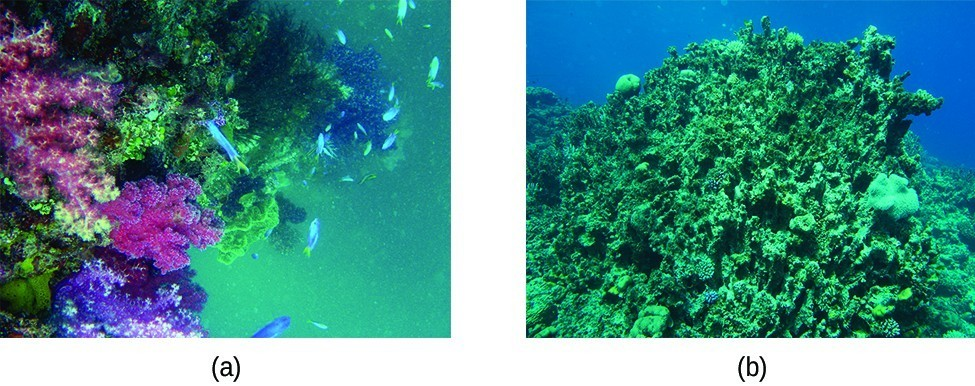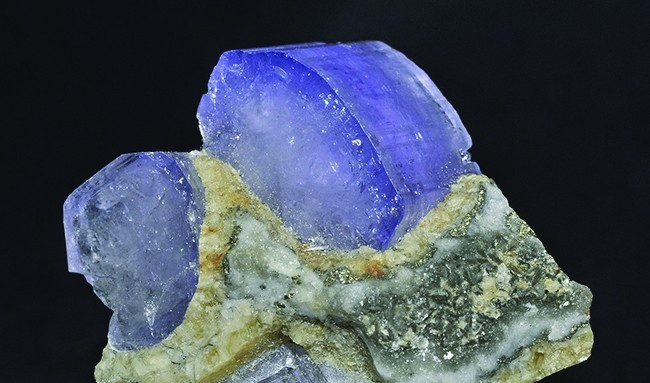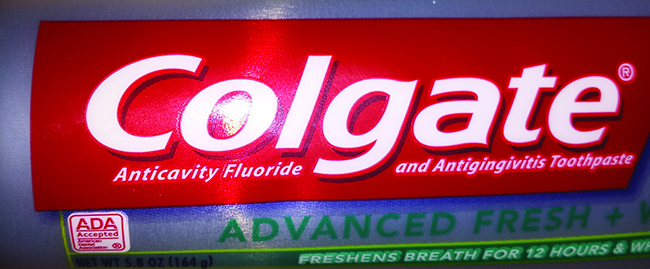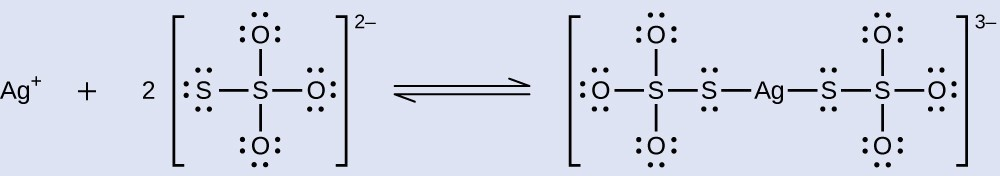## Multiple Equilibria

### Learning Objectives

By the end of this module, you will be able to:

• Describe examples of systems involving two (or more) simultaneous chemical equilibria
• Calculate reactant and product concentrations for multiple equilibrium systems
• Compare dissolution and weak electrolyte formation

There are times when one equilibrium reaction does not adequately describe the system being studied. Sometimes we have more than one type of equilibrium occurring at once (for example, an acid-base reaction and a precipitation reaction).

The ocean is a unique example of a system with multiple equilibria, or multiple states of solubility equilibria working simultaneously. Carbon dioxide in the air dissolves in sea water, forming carbonic acid (H2CO3). The carbonic acid then ionizes to form hydrogen ions and bicarbonate ions $\left({\text{HCO}}_{3}{}^{-}\right)$, which can further ionize into more hydrogen ions and carbonate ions $\left({\text{CO}}_{3}{}^{2-}\right):$

$\begin{array}{rll}{}{\text{CO}}_{2}\left(g\right)&\rightleftharpoons&{\text{CO}}_{2}\left(aq\right)\\{\text{CO}}_{2}\left(aq\right)+{\text{H}}_{2}\text{O}&\rightleftharpoons&{\text{H}}_{2}{\text{CO}}_{3}\left(aq\right)\\{\text{H}}_{2}{\text{CO}}_{3}\left(aq\right)&\rightleftharpoons&{\text{H}}^{\text{+}}\left(aq\right)+{\text{HCO}}_{3}{}^{-}\left(aq\right)\\{\text{HCO}}_{3}{}^{-}\left(aq\right)&\rightleftharpoons&{\text{H}}^{\text{+}}\left(aq\right)+{\text{CO}}_{3}{}^{2-}\left(aq\right)\end{array}$

The excess H+ ions make seawater more acidic. Increased ocean acidification can then have negative impacts on reef-building coral, as they cannot absorb the calcium carbonate they need to grow and maintain their skeletons (Figure 1). This in turn disrupts the local biosystem that depends upon the health of the reefs for its survival. If enough local reefs are similarly affected, the disruptions to sea life can be felt globally. The world’s oceans are presently in the midst of a period of intense acidification, believed to have begun in the mid-nineteenth century, and which is now accelerating at a rate faster than any change to oceanic pH in the last 20 million years.Figure 1. Healthy coral reefs (a) support a dense and diverse array of sea life across the ocean food chain. But when coral are unable to adequately build and maintain their calcium carbonite skeletons because of excess ocean acidification, the unhealthy reef (b) is only capable of hosting a small fraction of the species as before, and the local food chain starts to collapse. (credit a: modification of work by NOAA Photo Library; credit b: modification of work by “prilfish”/Flickr)

This site has detailed information about how ocean acidification specifically affects coral reefs.

Slightly soluble solids derived from weak acids generally dissolve in strong acids, unless their solubility products are extremely small. For example, we can dissolve CuCO3, FeS, and Ca3(PO4)2 in HCl because their basic anions react to form weak acids (H2CO3, H2S, and ${\text{H}}_{2}{\text{PO}}_{4}^{-}$ ). The resulting decrease in the concentration of the anion results in a shift of the equilibrium concentrations to the right in accordance with Le Châtelier’s principle.

Of particular relevance to us is the dissolution of hydroxylapatite, Ca5(PO4)3OH, in acid. Apatites are a class of calcium phosphate minerals (Figure 2); a biological form of hydroxylapatite is found as the principal mineral in the enamel of our teeth. A mixture of hydroxylapatite and water (or saliva) contains an equilibrium mixture of solid Ca5(PO4)3OH and dissolved Ca2+, ${\text{PO}}_{4}^{3-}$, and OH ions:

${\text{Ca}}_{5}{\left({\text{PO}}_{4}\right)}_{3}\text{OH}\left(s\right)\longrightarrow 5{\text{Ca}}^{\text{2+}}\left(aq\right)+3{\text{PO}}_{4}^{3-}\left(aq\right)+{\text{OH}}^{-}\left(aq\right)$Figure 2. Crystal of the mineral hydroxylapatite, Ca5(PO4)3OH, is shown here. Pure apatite is white, but like many other minerals, this sample is colored because of the presence of impurities.

When exposed to acid, phosphate ions react with hydronium ions to form hydrogen phosphate ions and ultimately, phosphoric acid:

${\text{PO}}_{4}{}^{3-}\left(aq\right)+{\text{H}}_{3}{\text{O}}^{+}\longrightarrow {\text{H}}_{2}{\text{PO}}_{4}{}^{2-}+{\text{H}}_{2}\text{O}$
${\text{PO}}_{4}{}^{2-}\left(aq\right)+{\text{H}}_{3}{\text{O}}^{+}\longrightarrow {\text{H}}_{2}{\text{PO}}_{4}{}^{-}+{\text{H}}_{2}\text{O}$
${\text{H}}_{2}{\text{PO}}_{4}{}^{-}+{\text{H}}_{3}{\text{O}}^{+}\longrightarrow {\text{H}}_{3}{\text{PO}}_{4}+{\text{H}}_{2}\text{O}$

Hydroxide ion reacts to form water:

${\text{OH}}^{-}\left(aq\right)+{\text{H}}_{3}{\text{O}}^{+}\longrightarrow 2{\text{H}}_{2}\text{O}$

These reactions decrease the phosphate and hydroxide ion concentrations, and additional hydroxylapatite dissolves in an acidic solution in accord with Le Châtelier’s principle. Our teeth develop cavities when acid waste produced by bacteria growing on them causes the hydroxylapatite of the enamel to dissolve. Fluoride toothpastes contain sodium fluoride, NaF, or stannous fluoride [more properly named tin(II) fluoride], SnF2. They function by replacing the OH ion in hydroxylapatite with F ion, producing fluorapatite, Ca5(PO4)3F:

$\text{NaF}+{\text{Ca}}_{5}{\left({\text{PO}}_{4}\right)}_{3}\text{OH}\rightleftharpoons {\text{Ca}}_{5}{\left({\text{PO}}_{4}\right)}_{3}\text{F}+{\text{Na}}^{+}+{\text{OH}}^{-}$

The resulting Ca5(PO4)3F is slightly less soluble than Ca5(PO4)3OH, and F is a weaker base than OH. Both of these factors make the fluorapatite more resistant to attack by acids than hydroxylapatite. See the Chemistry in Everyday Life feature on the role of fluoride in preventing tooth decay for more information.

### Role of Fluoride in Preventing Tooth Decay

As we saw previously, fluoride ions help protect our teeth by reacting with hydroxylapatite to form fluorapatite, Ca5(PO4)3F. Since it lacks a hydroxide ion, fluorapatite is more resistant to attacks by acids in our mouths and is thus less soluble, protecting our teeth. Scientists discovered that naturally fluorinated water could be beneficial to your teeth, and so it became common practice to add fluoride to drinking water. Toothpastes and mouthwashes also contain amounts of fluoride (Figure 3).Figure 3. Fluoride, found in many toothpastes, helps prevent tooth decay (credit: Kerry Ceszyk).

Unfortunately, excess fluoride can negate its advantages. Natural sources of drinking water in various parts of the world have varying concentrations of fluoride, and places where that concentration is high are prone to certain health risks when there is no other source of drinking water. The most serious side effect of excess fluoride is the bone disease, skeletal fluorosis. When excess fluoride is in the body, it can cause the joints to stiffen and the bones to thicken. It can severely impact mobility and can negatively affect the thyroid gland. Skeletal fluorosis is a condition that over 2.7 million people suffer from across the world. So while fluoride can protect our teeth from decay, the US Environmental Protection Agency sets a maximum level of 4 ppm (4 mg/L) of fluoride in drinking water in the US. Fluoride levels in water are not regulated in all countries, so fluorosis is a problem in areas with high levels of fluoride in the groundwater.

When acid rain attacks limestone or marble, which are calcium carbonates, a reaction occurs that is similar to the acid attack on hydroxylapatite. The hydronium ion from the acid rain combines with the carbonate ion from calcium carbonates and forms the hydrogen carbonate ion, a weak acid:

${\text{H}}_{3}{\text{O}}^{\text{+}}\left(aq\right)+{\text{CO}}_{3}{}^{2-}\left(aq\right)\longrightarrow {\text{HCO}}_{3}{}^{-}\left(aq\right)+{\text{H}}_{2}\text{O}\left(l\right)$

Calcium hydrogen carbonate, Ca(HCO3)2, is soluble, so limestone and marble objects slowly dissolve in acid rain.

If we add calcium carbonate to a concentrated acid, hydronium ion reacts with the carbonate ion according to the equation:

$2{\text{H}}_{3}{\text{O}}^{\text{+}}\left(aq\right)+{\text{CO}}_{3}{}^{2-}\left(aq\right)\longrightarrow {\text{H}}_{2}{\text{CO}}_{3}\left(aq\right)+2{\text{H}}_{2}\text{O}\left(l\right)$

(Acid rain is usually not sufficiently acidic to cause this reaction; however, laboratory acids are.) The solution may become saturated with the weak electrolyte carbonic acid, which is unstable, and carbon dioxide gas can be evolved:

${\text{H}}_{2}{\text{CO}}_{3}\left(aq\right)\longrightarrow {\text{CO}}_{2}\left(g\right)+{\text{H}}_{2}\text{O}\left(l\right)$

These reactions decrease the carbonate ion concentration, and additional calcium carbonate dissolves. If enough acid is present, the concentration of carbonate ion is reduced to such a low level that the reaction quotient for the dissolution of calcium carbonate remains less than the solubility product of calcium carbonate, even after all of the calcium carbonate has dissolved.

### Example 1: Prevention of Precipitation of Mg(OH)2

Calculate the concentration of ammonium ion that is required to prevent the precipitation of Mg(OH)2 in a solution with [Mg2+] = 0.10 M and [NH3] = 0.10 M.

Consider the two equilibria:

$\text{ZnS}\left(s\right)\rightleftharpoons {\text{Zn}}^{\text{2+}}\left(aq\right)+{\text{S}}^{2-}\left(aq\right);{K}_{\text{sp}}=1\times {10}^{-27}$
$2{\text{H}}_{2}\text{O}\left(l\right)+{\text{H}}_{2}\text{S}\left(aq\right)\rightleftharpoons 2{\text{H}}_{3}{\text{O}}^{\text{+}}\left(aq\right)+{\text{S}}^{2-}\left(aq\right);K=1.0\times {10}^{\text{-26}}$

and calculate the concentration of hydronium ion required to prevent the precipitation of ZnS in a solution that is 0.050 M in Zn2+ and saturated with H2S (0.10 M H2S).

Therefore, precise calculations of the solubility of solids from the solubility product are limited to cases in which the only significant reaction occurring when the solid dissolves is the formation of its ions.

### Example 2: Multiple Equilibria

Unexposed silver halides are removed from photographic film when they react with sodium thiosulfate (Na2S2O3, called hypo) to form the complex ion $\text{Ag}{\left({\text{S}}_{2}{\text{O}}_{3}\right)}_{2}{}^{3-}$ (Kf = 4.7 $\times$ 1013). The reaction with silver bromide is:What mass of Na2S2O3 is required to prepare 1.00 L of a solution that will dissolve 1.00 g of AgBr by the formation of $\text{Ag}{\left({\text{S}}_{2}{\text{O}}_{3}\right)}_{2}{}^{3-}?$

AgCl(s), silver chloride, is well known to have a very low solubility: Ag(s) ⇌ Ag+(aq) + Cl(aq), Ksp = 1.77 × 10–10. Adding ammonia significantly increases the solubility of AgCl because a complex ion is formed: Ag+(aq) + 2NH3(aq) ⇌ Ag(NH3)2+(aq), Kf = 1.7 × 107. What mass of NH3 is required to prepare 1.00 L of solution that will dissolve 2.00 g of AgCl by formation of Ag(NH3)2+?

## Dissolution versus Weak Electrolyte Formation

We can determine how to shift the concentration of ions in the equilibrium between a slightly soluble solid and a solution of its ions by applying Le Châtelier’s principle. For example, one way to control the concentration of manganese(II) ion, Mn2+, in a solution is to adjust the pH of the solution and, consequently, to manipulate the equilibrium between the slightly soluble solid manganese(II) hydroxide, manganese(II) ion, and hydroxide ion:

$\text{Mn}{\left(\text{OH}\right)}_{2}\left(s\right)\rightleftharpoons {\text{Mn}}^{\text{2+}}\left(aq\right)+2{\text{OH}}^{-}\left(aq\right){K}_{\text{sp}}=\left[{\text{Mn}}^{\text{2+}}\right]{\left[{\text{OH}}^{-}\right]}^{2}$

This could be important to a laundry because clothing washed in water that has a manganese concentration exceeding 0.1 mg per liter may be stained by the manganese. We can reduce the concentration of manganese by increasing the concentration of hydroxide ion. We could add, for example, a small amount of NaOH or some other base such as the silicates found in many laundry detergents. As the concentration of OH ion increases, the equilibrium responds by shifting to the left and reducing the concentration of Mn2+ ion while increasing the amount of solid Mn(OH)2 in the equilibrium mixture, as predicted by Le Châtelier’s principle.

### Example 3: Solubility Equilibrium of a Slightly Soluble Solid

What is the effect on the amount of solid Mg(OH)2 that dissolves and the concentrations of Mg2+ and OH when each of the following are added to a mixture of solid Mg(OH)2 in water at equilibrium?

1. MgCl2
2. KOH
3. an acid
4. NaNO3
5. Mg(OH)2

What is the effect on the amount of solid NiCO3 that dissolves and the concentrations of Ni2+ and ${\text{CO}}_{3}{}^{2-}$ when each of the following are added to a mixture of the slightly soluble solid NiCO3 and water at equilibrium?

1. Ni(NO3)2
2. KClO4
3. NiCO3
4. K2CO3
5. HNO3 (reacts with carbonate giving ${\text{HCO}}_{3}{}^{-}$ or H2O and CO2)

### Key Concepts and Summary

Several systems we encounter consist of multiple equilibria, systems where two or more equilibria processes are occurring simultaneously. Some common examples include acid rain, fluoridation, and dissolution of carbon dioxide in sea water. When looking at these systems, we need to consider each equilibrium separately and then combine the individual equilibrium constants into one solubility product or reaction quotient expression using the tools from the first equilibrium chapter. Le Châtelier’s principle also must be considered, as each reaction in a multiple equilibria system will shift toward reactants or products based on what is added to the initial reaction and how it affects each subsequent equilibrium reaction.

### Exercises

1. A saturated solution of a slightly soluble electrolyte in contact with some of the solid electrolyte is said to be a system in equilibrium. Explain. Why is such a system called a heterogeneous equilibrium?
2. Calculate the equilibrium concentration of Ni2+ in a 1.0-M solution [Ni(NH3)6](NO3)2.
3. Calculate the equilibrium concentration of Zn2+ in a 0.30-M solution of $\text{Zn}{\left(\text{CN}\right)}_{4}{}^{2-}$.
4. Calculate the equilibrium concentration of Cu2+ in a solution initially with 0.050 M Cu2+ and 1.00 M NH3.
5. Calculate the equilibrium concentration of Zn2+ in a solution initially with 0.150 M Zn2+ and 2.50 M CN.
6. Calculate the Fe3+ equilibrium concentration when 0.0888 mole of K3[Fe(CN)6] is added to a solution with 0.0.00010 M CN.
7. Calculate the Co2+ equilibrium concentration when 0.100 mole of [Co(NH3)6](NO3)2 is added to a solution with 0.025 M NH3. Assume the volume is 1.00 L.
8. The equilibrium constant for the reaction ${\text{Hg}}^{\text{2+}}\left(aq\right)+2{\text{Cl}}^{-}\left(aq\right)\rightleftharpoons {\text{HgCl}}_{2}\left(aq\right)$ is 1.6 $\times$ 1013. Is HgCl2 a strong electrolyte or a weak electrolyte? What are the concentrations of Hg2+ and Cl in a 0.015-M solution of HgCl2?
9. Calculate the molar solubility of Sn(OH)2 in a buffer solution containing equal concentrations of NH3 and ${\text{NH}}_{4}{}^{+}$.
10. Calculate the molar solubility of Al(OH)3 in a buffer solution with 0.100 M NH3 and 0.400 M ${\text{NH}}_{4}{}^{+}$.
11. What is the molar solubility of CaF2 in a 0.100-M solution of HF? Ka for HF = 7.2 $\times$ 10–4.
12. What is the molar solubility of BaSO4 in a 0.250-M solution of NaHSO4? Ka for ${\text{HSO}}_{4}{}^{-}$ = 1.2 $\times$ 10–2.
13. What is the molar solubility of Tl(OH)3 in a 0.10-M solution of NH3?
14. What is the molar solubility of Pb(OH)2 in a 0.138-M solution of CH3NH2?
15. A solution of 0.075 M CoBr2 is saturated with H2S ([H2S] = 0.10 M). What is the minimum pH at which CoS begins to precipitate?
$\text{CoS}\left(s\right)\rightleftharpoons {\text{Co}}^{\text{2+}}\left(aq\right)+{\text{S}}^{2-}\left(aq\right){K}_{\text{sp}}=4.5\times {10}^{-27}$${\text{H}}_{2}\text{S}\left(aq\right)+2{\text{H}}_{2}\text{O}\left(l\right)\rightleftharpoons 2{\text{H}}_{3}{\text{O}}^{\text{+}}\left(aq\right)+{\text{S}}^{2-}\left(aq\right)K=1.0\times {10}^{\text{-26}}$
16. A 0.125-M solution of Mn(NO3)2 is saturated with H2S ([H2S] = 0.10 M). At what pH does MnS begin to precipitate?$\text{MnS}\left(s\right)\rightleftharpoons {\text{Mn}}^{\text{2+}}\left(aq\right)+{\text{S}}^{2-}\left(aq\right){K}_{\text{sp}}=4.3\times {10}^{-22}$${\text{H}}_{2}\text{S}\left(aq\right)+2{\text{H}}_{2}\text{O}\left(l\right)\rightleftharpoons 2{\text{H}}_{3}{\text{O}}^{\text{+}}\left(aq\right)+{\text{S}}^{2-}\left(aq\right)K=1.0\times {10}^{-26}$
17. Calculate the molar solubility of BaF2 in a buffer solution containing 0.20 M HF and 0.20 M NaF.
18. Calculate the molar solubility of CdCO3 in a buffer solution containing 0.115 M Na2CO3 and 0.120 M NaHCO3
19. To a 0.10-M solution of Pb(NO3)2 is added enough HF(g) to make [HF] = 0.10 M.
1. Does PbF2 precipitate from this solution? Show the calculations that support your conclusion.
2. What is the minimum pH at which PbF2 precipitates?
20. Calculate the concentration of Cd2+ resulting from the dissolution of CdCO3 in a solution that is 0.250 M in CH3CO2H, 0.375 M in NaCH3CO2, and 0.010 M in H2CO3.
21. Both AgCl and AgI dissolve in NH3.
1. What mass of AgI dissolves in 1.0 L of 1.0 M NH3?
2. What mass of AgCl dissolves in 1.0 L of 1.0 M NH3?
22. Calculate the volume of 1.50 M CH3CO2H required to dissolve a precipitate composed of 350 mg each of CaCO3, SrCO3, and BaCO3.
23. Even though Ca(OH)2 is an inexpensive base, its limited solubility restricts its use. What is the pH of a saturated solution of Ca(OH)2?
24. What mass of NaCN must be added to 1 L of 0.010 M Mg(NO3)2 in order to produce the first trace of Mg(OH)2?
25. Magnesium hydroxide and magnesium citrate function as mild laxatives when they reach the small intestine. Why do magnesium hydroxide and magnesium citrate, two very different substances, have the same effect in your small intestine. (Hint: The contents of the small intestine are basic.)
26. The following question is taken from a Chemistry Advanced Placement Examination and is used with the permission of the Educational Testing Service. Solve the following problem: ${\text{MgF}}_{2}\left(s\right)\rightleftharpoons {\text{Mg}}^{\text{2+}}\left(aq\right)+2{\text{F}}^{-}\left(aq\right)$In a saturated solution of MgF2 at 18 °C, the concentration of Mg2+ is 1.21 $\times$ 10–3M. The equilibrium is represented by the equation above.
1. Write the expression for the solubility-product constant, Ksp, and calculate its value at 18 °C.
2. Calculate the equilibrium concentration of Mg2+ in 1.000 L of saturated MgF2 solution at 18 °C to which 0.100 mol of solid KF has been added. The KF dissolves completely. Assume the volume change is negligible.
3. Predict whether a precipitate of MgF2 will form when 100.0 mL of a 3.00 $\times$ 10–3M solution of Mg(NO3)2 is mixed with 200.0 mL of a 2.00 $\times$ 10–3M solution of NaF at 18 °C. Show the calculations to support your prediction.
4. At 27 °C the concentration of Mg2+ in a saturated solution of MgF2 is 1.17 $\times$ 10–3M. Is the dissolving of MgF2 in water an endothermic or an exothermic process? Give an explanation to support your conclusion.
27. Which of the following compounds, when dissolved in a 0.01-M solution of HClO4, has a solubility greater than in pure water: AgBr, BaF2, Ca3(PO4)3, ZnS, PbI2? Explain your answer.
28. What is the effect on the amount of solid Mg(OH)2 that dissolves and the concentrations of Mg2+ and OH when each of the following are added to a mixture of solid Mg(OH)2 and water at equilibrium?
1. MgCl2
2. KOH
3. HClO4
4. NaNO3
5. Mg(OH)2
29. What is the effect on the amount of CaHPO4 that dissolves and the concentrations of Ca2+ and ${\text{HPO}}_{4}{}^{-}$ when each of the following are added to a mixture of solid CaHPO4 and water at equilibrium?
1. CaCl2
2. HCl
3. KClO4
4. NaOH
5. CaHPO4
30. Identify all chemical species present in an aqueous solution of Ca3(PO4)2 and list these species in decreasing order of their concentrations. (Hint: Remember that the ${\text{PO}}_{4}{}^{\text{3-}}$ ion is a weak base.)
31. A volume of 50 mL of 1.8 M NH3 is mixed with an equal volume of a solution containing 0.95 g of MgCl2. What mass of NH4Cl must be added to the resulting solution to prevent the precipitation of Mg(OH)2?

## Glossary

multiple equilibrium: system characterized by more than one state of balance between a slightly soluble ionic solid and an aqueous solution of ions working simultaneously